# How to compare two Excel sheets using Vlookup

You can watch a video tutorial here.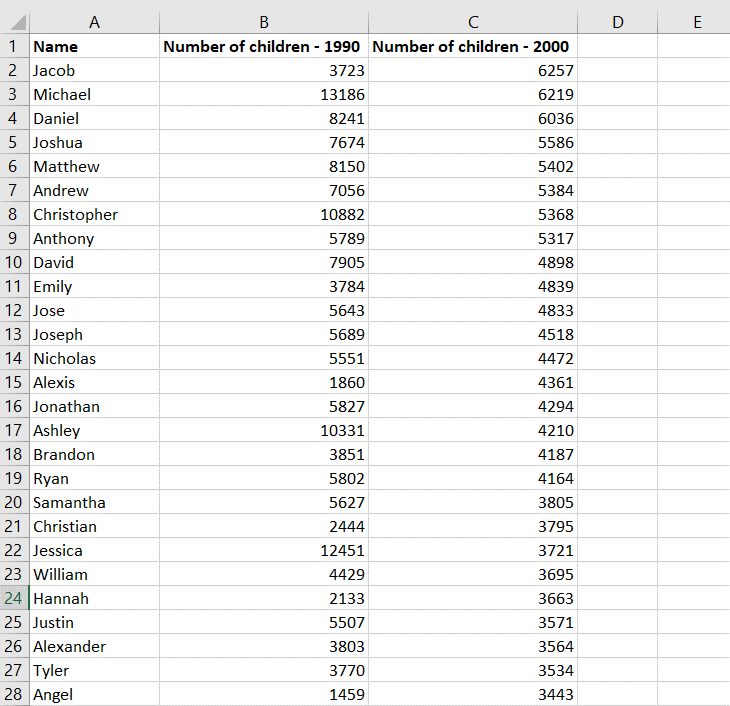Vlookup is one of the most useful functions in Excel when it comes to comparing data from multiple sheets. As long as the sheets share a column field or ‘key’, data from both sheets can be easily compared. In this example, we will compare the popularity of baby names in 1990 to that in the year 2000. Here the key is the name.

### Step 1 – Identify the key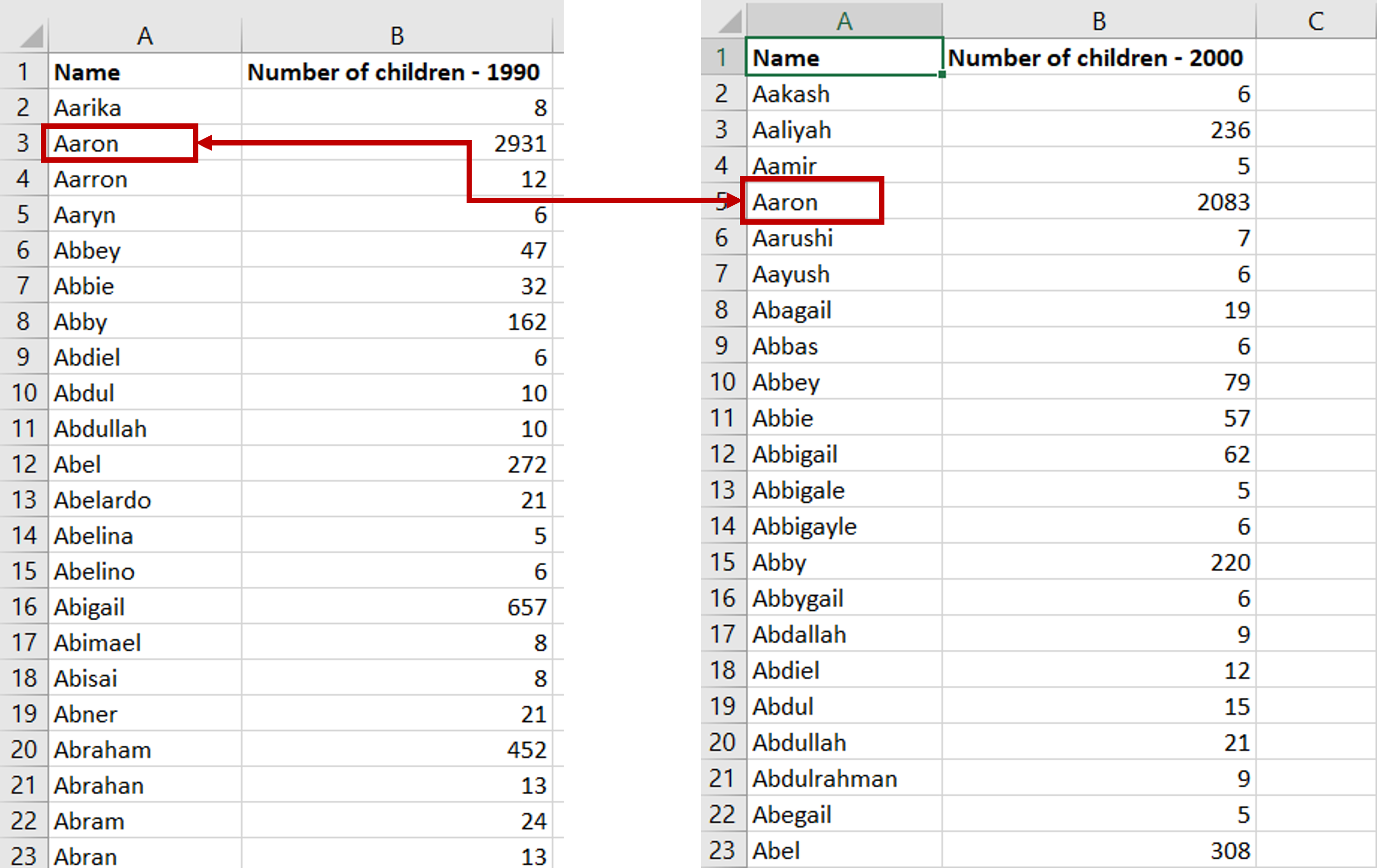– The key is the column ‘Name’
– The data from 1990 will be Table 1 and the data from 2000 will be Table 2
Note: A few things to keep in mind when using Vlookup:
The key must always be in the left-most column of Table 2
– Table 2, or the table that is being referred to must always be sorted on the key
It is better Table 2 has unique values or else this function may not return the desired result

### Step 2 – Open the Formula wizard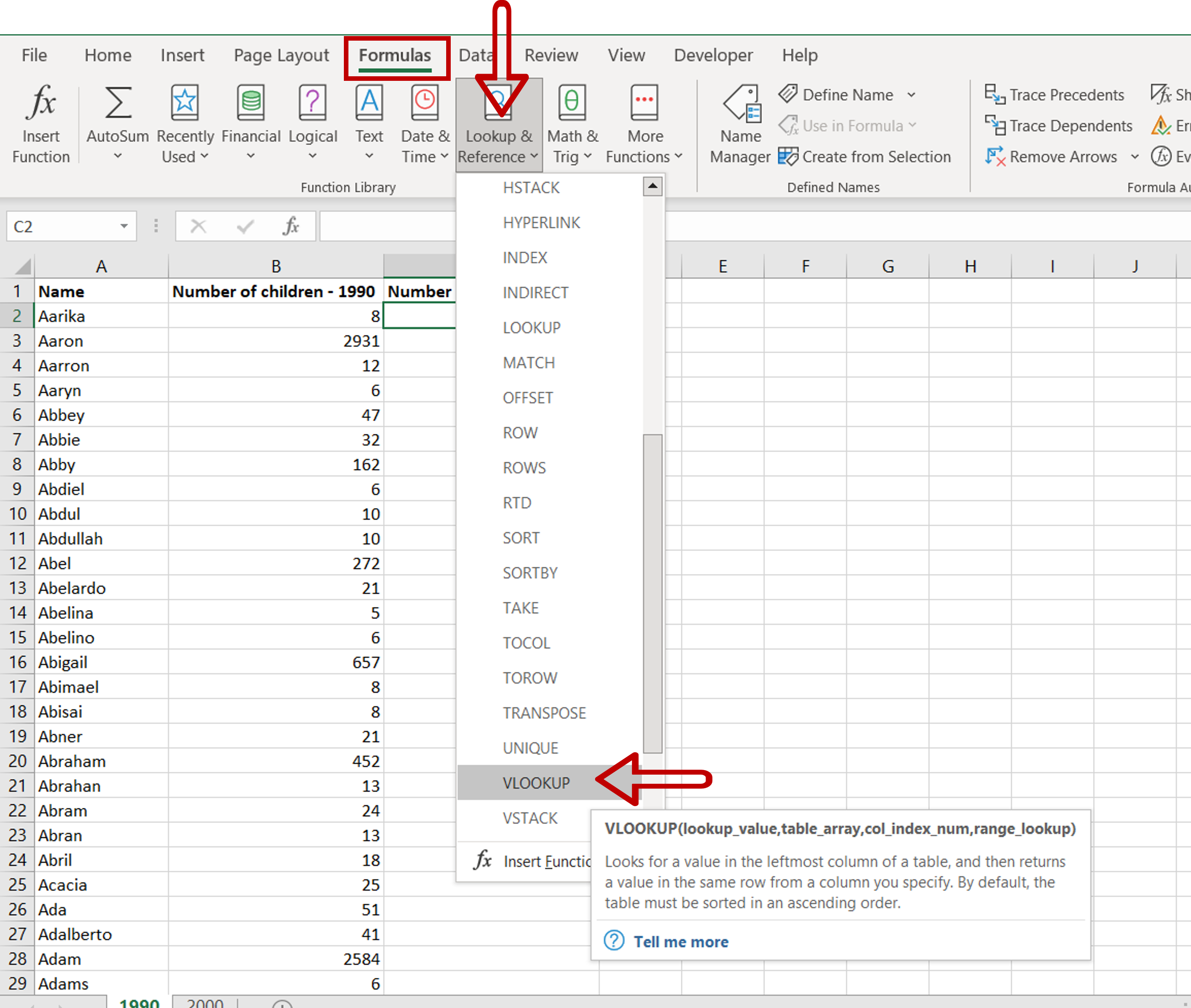– Go to the Formulas > Lookup & Reference > Vlookup

### Step 3 – Enter the arguments for the VLOOKUP() function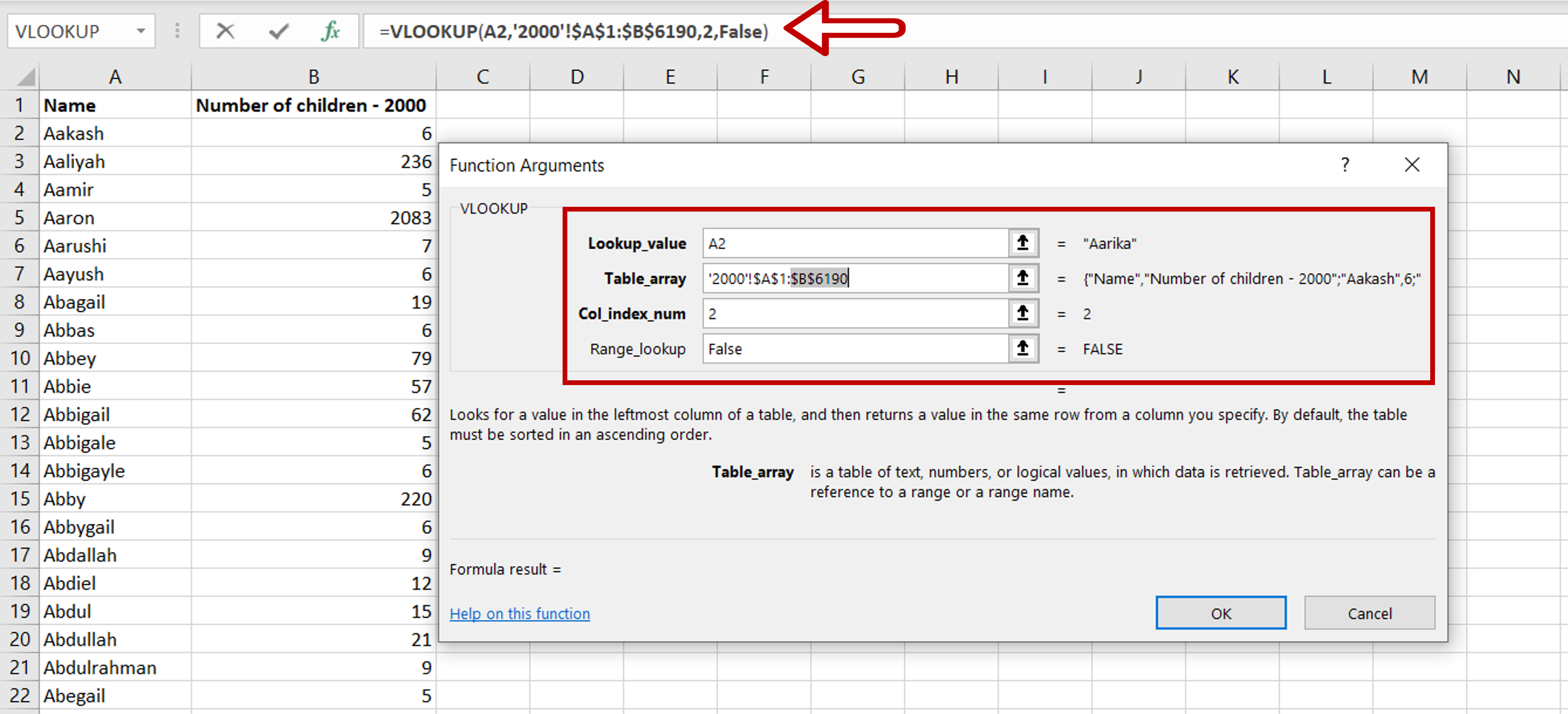Lookup value: the cell reference of the first value in the key column from Table 1 (Name)
– Table-array: the range of Table 2 which is made constant with the addition of dollar signs (\$)
– Col_index_num: the number of the column in Table 2 that has to be retrieved (2 i.e. Number of children – 2000)
– Range_lookup: TRUE will return an approximate match, FALSE will return an exact match
– Click OK

### Step 4 – Enhance the formula to handle errors i.e. missing values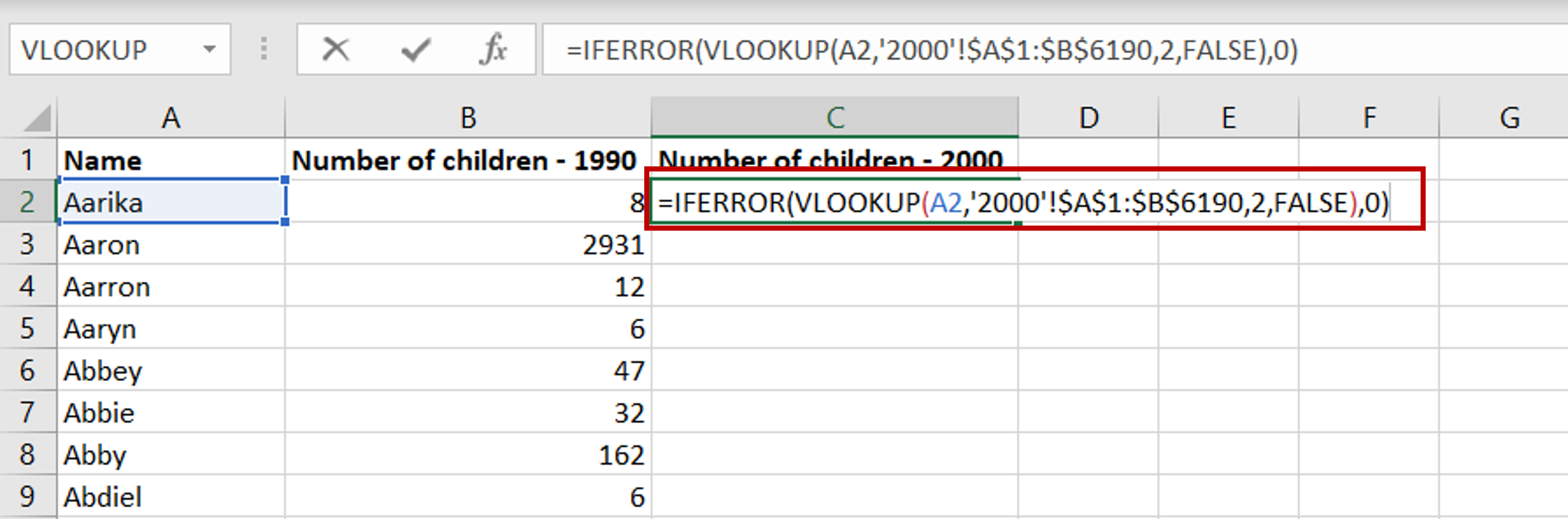– If the lookup function cannot find a match in Table 2 i.e. the name is not present in Table 2, an error message is returned  – #N/A
– Change the formula to display zero (0) if a match cannot be found:
= iferror(<vlookup formula>,0)

### Step 5 – Copy the formula– Using the fill handle from the first cell, drag the formula to the remaining cells
OR
a) Select the cell with the formula and press Ctrl+C or choose Copy from the context menu (right-click)
b) Select the rest of the cells in the column and press Ctrl+V or choose Paste from the context menu (right-click)

### Step 6 – Sort the data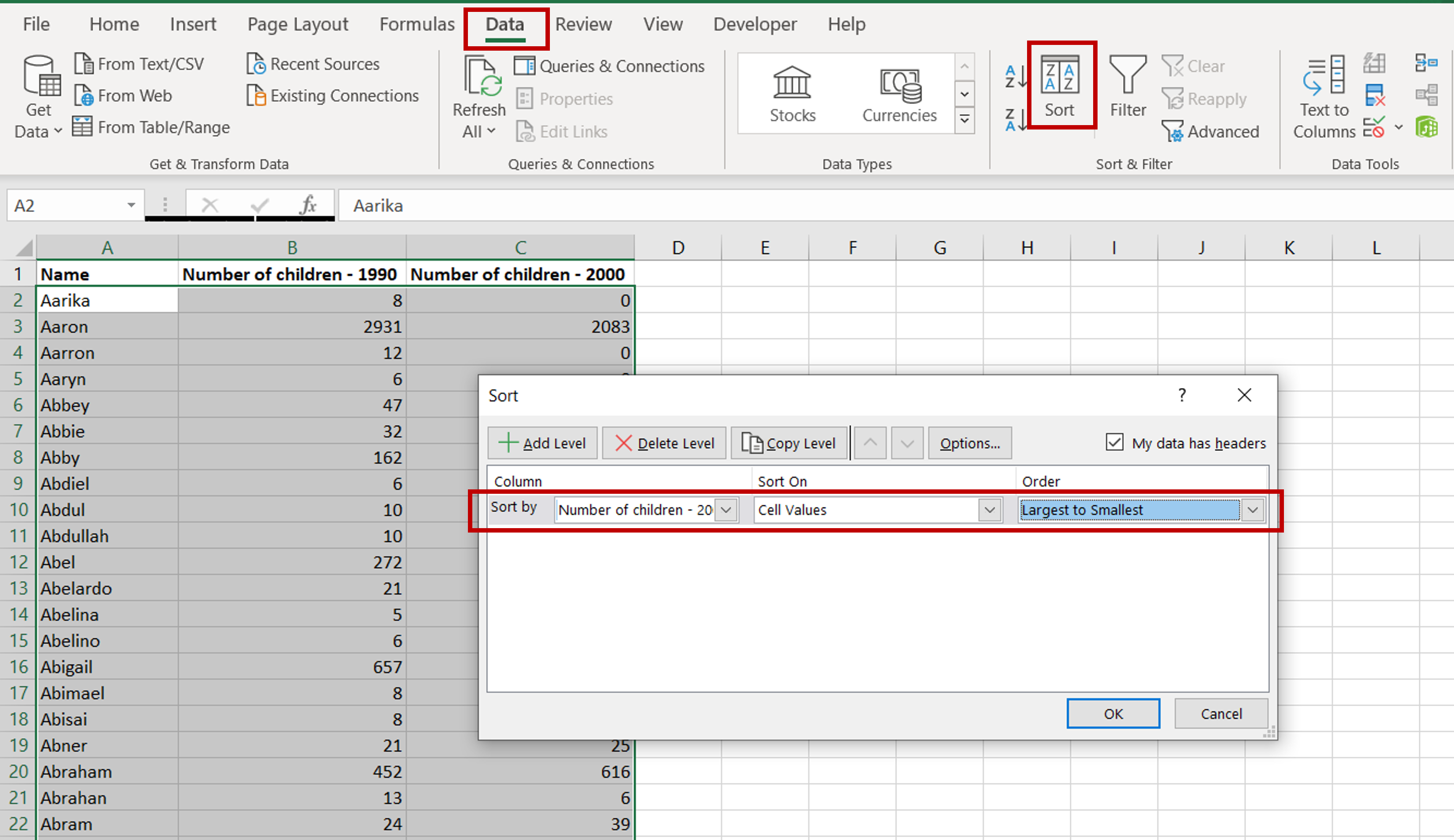– Go to Data > Sort
– Select ‘Number of children – 2000’ under Sort by
– Choose ‘Largest to Smallest’ under Order
– Click OK

### Step 7 – Check the data– The data is ready to be compared